# Pre-Algebra : Negative Numbers

## Example Questions

### Example Question #1 : Subtract Rational Numbers And Understand The Absolute Value Of Their Difference: Ccss.Math.Content.7.Ns.A.1c

Subtract: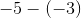Explanation:

Distribute the negative sign through the parentheses.  Double negatives equal a positive.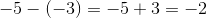The answer is.

### Example Question #31 : Negative Numbers

Solve: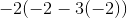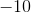Explanation:

To evaluate this, first simplify the innermost parentheses.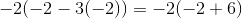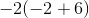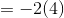Distribute the negative two in front to term inside the parentheses.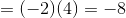### Example Question #1 : Add And Subtract Rational Numbers: Ccss.Math.Content.7.Ns.A.1d

Evaluate: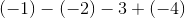Explanation:

Break up the parentheses. Double negatives result in a positive sign, and positive with a negative sign will result in a negative sign.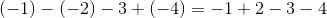Next, simplify by adding and subtracting each component from left to right.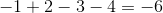### Example Question #32 : Negative Numbers

Solve: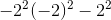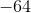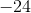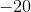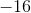Explanation:

Use the order of operations to solve this.  A negative number that is not in parentheses squared will remain negative.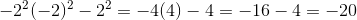### Example Question #31 : Negative Numbers

Simplify: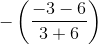Explanation:

Simplify the numerator and the denominator first.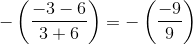Divide the terms inside the parentheses.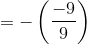Distribute the negative sign.  Double negatives will result in a positive sign.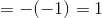### Example Question #41 : The Number System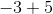Explanation:

Sinceis greater thanand is positive, our answer is positive. We treat as a subtraction problem. Answer is.

### Example Question #8 : Understand Distances Between Numbers On A Number Line: Ccss.Math.Content.7.Ns.A.1b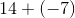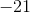Explanation:

When a plus and minus sign meet, the sign is negative. The difference is.

### Example Question #41 : The Number System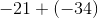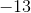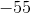Explanation:

When a plus and a minus meet, the sign is negative. When adding two negatie numbers, we treat as addition and add the minus sign in the end. Answer is.

### Example Question #5 : Subtract Rational Numbers And Understand The Absolute Value Of Their Difference: Ccss.Math.Content.7.Ns.A.1c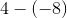Explanation:

When minus signs meet, the sign becomes positive. This becomes an addition problem. Answer is.

### Example Question #34 : Negative Numbers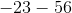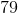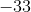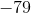This is also the sum of two negative numbers. In this case, we add the numbers and then add a minus sign in the end. Answer is.